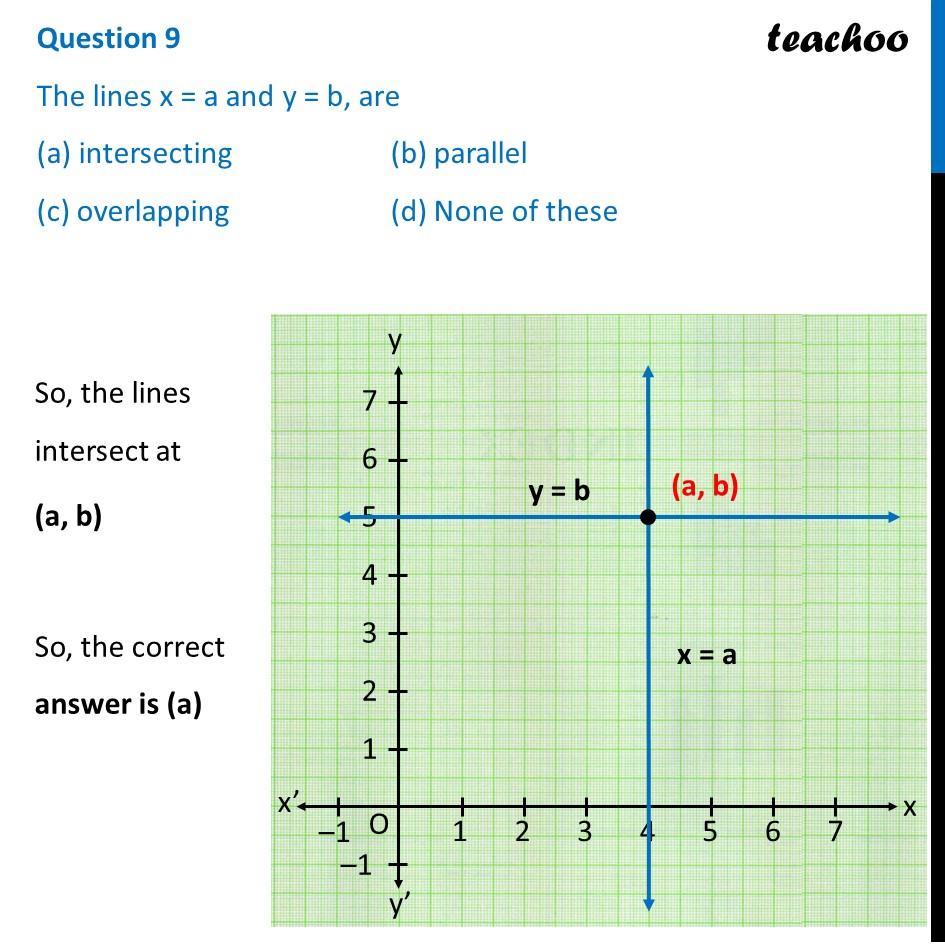## (c) overlapping   (d) None of these

This question is inspired from Question 7 - MCQs from NCERT Exemplar - Chapter 3 Class 10 - Pair of Linear Equations in Two Variables1. Class 10
2. Solutions of Sample Papers for Class 10 Boards
3. CBSE Class 10 Sample Paper for 2022 Boards - Maths Standard [MCQ]

Transcript

Question 9 The lines x = a and y = b, are (a) intersecting (b) parallel (c) overlapping (d) None of these So, the lines intersect at (a, b) So, the correct answer is (a)